## Burnside Problem

A problem originating with W. Burnside (1902), who wrote, A still undecided point in the theory of discontinuous groups is whether the Order of a Group may be not finite, while the order of every operation it contains is finite.'' This question would now be phrased as Can a finitely generated group be infinite while every element in the group has finite order?'' (Vaughan-Lee 1990). This question was answered by Golod (1964) when he constructed finitely generated infinite p-Group. These Groups, however, do not have a finite exponent.

Letbe the Free Group of Rankand letbe the Subgroup generated by the set ofth Powers. Thenis a normal subgroup of. We define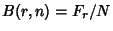to be the Quotient Group. We callthe-generator Burnside group of exponent. It is the largest-generator group of exponent, in the sense that every other such group is a Homeomorphic image of. The Burnside problem is usually stated as: For which values ofandisa Finite Group?''

An answer is known for the following values. For,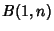is a Cyclic Group of Order. For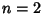,is an elementary Abelian 2-group of Order. For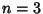,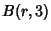was proved to be finite by Burnside. The Order of thegroups was established by Levi and van der Waerden (1933), namelywhere(1)

whereis a Binomial Coefficient. For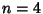,was proved to be finite by Sanov (1940). Groups of exponent four turn out to be the most complicated for which a Positive solution is known. The precise nilpotency class and derived length are known, as are bounds for the Order. For example,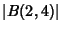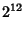(2)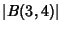(3)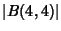(4)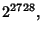(5)

while for larger values ofthe exact value is not yet known. For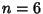,was proved to be finite by Hall (1958) with Order, where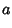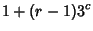(6)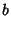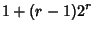(7)(8)

No other Burnside groups are known to be finite. On the other hand, for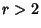and, withOdd,is infinite (Novikov and Adjan 1968). There is a similar fact foranda large Power of 2.

E. Zelmanov was awarded a Fields Medal in 1994 for his solution of the restricted'' Burnside problem.

References

Burnside, W. On an Unsettled Question in the Theory of Discontinuous Groups.'' Quart. J. Pure Appl. Math. 33, 230-238, 1902.

Golod, E. S. On Nil-Algebras and Residually Finite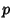-Groups.'' Isv. Akad. Nauk SSSR Ser. Mat. 28, 273-276, 1964.

Hall, M. Solution of the Burnside Problem for Exponent Six.'' Ill. J. Math. 2, 764-786, 1958.

Levi, F. and van der Waerden, B. L. Über eine besondere Klasse von Gruppen.'' Abh. Math. Sem. Univ. Hamburg 9, 154-158, 1933.

Novikov, P. S. and Adjan, S. I. Infinite Periodic Groups I, II, III.'' Izv. Akad. Nauk SSSR Ser. Mat. 32, 212-244, 251-524, and 709-731, 1968.

Sanov, I. N. Solution of Burnside's problem for exponent four.'' Leningrad State Univ. Ann. Math. Ser. 10, 166-170, 1940.

Vaughan-Lee, M. The Restricted Burnside Problem, 2nd ed. New York: Clarendon Press, 1993.